# Formula for Angle Range of Equal Circles Colliding?

##### New member
Hello. Thanks for having this forum. I'm long out of school, but I'd still like to know how to find the angles shown in my diagram. This has to do with billiard balls, but since the game is played on a single plane, it would work for 2D circles such as those shown. If I know the diameter or radius of the equal-size circles, then as distance changes between their centers, how can I determine how the angle range changes?

Shown in the diagram is a white cue ball below a shaded object ball. The crossed lines show the extreme outside points on the circles' surfaces where they would contact during collision. The vectors passing through the centers of the object balls are the post collision paths (perpendicular to the crossed lines) that the object balls would take for those contact points.
Essentially, the closer the balls are to one another, the smaller the angle range.

Does anyone know a formula to determine angles such as A, B or C in the diagram?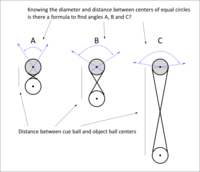#### MarkFL

##### Super Moderator
Staff member
I would begin by imposing one circle centered at the origin of an $$xy$$-plane, and another centered at the point $$(r,0)$$, where $$2R<r$$. Both circles have a radius of $$R$$.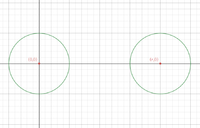Now, let's find the line that passes through the point $$\displaystyle \left(\frac{r}{2},0\right)$$, and which is tangent to the left circle. By symmetry, it will be tangent to the other circle as well. The line may be given as:

$$\displaystyle y=m\left(x-\frac{r}{2}\right)$$

Substituting this into the equation for the left circle, we obtain:

$$\displaystyle x^2+\left(m\left(x-\frac{r}{2}\right)\right)^2=R^2$$

Arrange this in standard quadratic form:

$$\displaystyle 4(m^2+1)x^2-4m^2rx+(m^2r^2-4R^2)=0$$

Equate the discriminant to zero:

$$\displaystyle (-4m^2r)^2-4(4(m^2+1))(m^2r^2-4R^2)=0$$

$$\displaystyle (m^2r)^2-(m^2+1)(m^2r^2-4R^2)=0$$

$$\displaystyle m^4r^2-m^4r^2+4m^2R^2-m^2r^2+4R^2=0$$

$$\displaystyle (4R^2-r^2)m^2+4R^2=0$$

$$\displaystyle m^2=\frac{4R^2}{r^2-4R^2}$$

Let's take the positive root:

$$\displaystyle m=\frac{2R}{\sqrt{r^2-4R^2}}$$

And so, our tangent line is given by:

$$\displaystyle y=\frac{2R}{\sqrt{r^2-4R^2}}\left(x-\frac{r}{2}\right)$$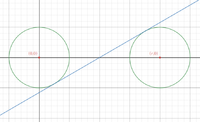Now, we are interested in the slope of a line perpendicular to this tangent line:

$$\displaystyle m_1=-\frac{\sqrt{r^2-4R^2}}{2R}$$

And so, the angle we desire will be:

$$\displaystyle \theta=2\arctan\left(\frac{\sqrt{r^2-4R^2}}{2R}\right)$$

•Denis

##### New member
Mark, never in all of my years on forums have I received such a quick, detailed and accurate reply. Thank you so much! I've studied your post for the past half hour, and I'm understanding much but not all. I'll study it some more. I even punched in extreme values to test it, and as of course you would know, it holds up beautifully. I loved math in grade school, and I'm beginning to regret not continuing to take it further. Fun stuff!

•MarkFL

#### MarkFL

##### Super Moderator
Staff member
Yes, I did examine the boundaries to make sure:

$$\displaystyle \lim_{r\to2R}\theta=0$$

$$\displaystyle \lim_{r\to\infty}\theta=\pi$$

Please feel free to ask about any parts of the derivation that aren't clear. I skipped some of the details, assuming you were ultimately after a working formula.#### LCKurtz

##### Full Member
Here's another way to look at the problem of finding the angle $$\displaystyle \theta$$. Consider the following picture: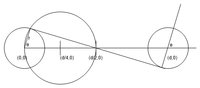The right triangle is inscribed in the circle with diameter $$\displaystyle \frac d 2$$ centered at $$\displaystyle \frac d 4$$. You can get $$\displaystyle \theta$$ from $$\displaystyle \cos\theta = \frac r {\frac d 2} = \frac {2r} d = \frac D d$$ where $$\displaystyle D$$ is the diameter of the ball. All you need is the diameter of the ball and the distance between their centers.

•MarkFL

##### New member
Interesting, LCKurtz.
So the angle that represents half of the range I'm seeking is arccosine=D/d
?

#### LCKurtz

##### Full Member
Interesting, LCKurtz.
So the angle that represents half of the range I'm seeking is arccosine=D/d
?
Yes.

#### Dr.Peterson

##### Elite Member
Interesting, LCKurtz.
So the angle that represents half of the range I'm seeking is arccosine=D/d
?
You mean arccos(D/d), right?

#### LCKurtz

##### Full Member
@grimlad: If you want another little challenge see if you can modify my argument to get the answer if the balls have different radii, say $$\displaystyle r$$ and $$\displaystyle R$$. You might be surprised how similar and simple it is.

##### New member
I'm visualizing the ball sizes changing as your lines move, but I'm just not equipped to figure it out.##### New member
Here’s a related question if you wouldn’t mind. My original question was to find the range for all shots left, right and straight, and therefore theta could have been a maximum of 180 degrees. For this new question, let’s work only on the left side of that range, with theta being a maximum of 90 degrees---0 degrees being a shot where the cue ball strikes the object ball full in the face and the object ball releases from collision along a 0 degree path straight ahead, and 90 degrees being an impossible thin cut shot where the object ball is barely grazed at the right side and releases from collision along a path directly to the left.

A path of 0 degrees is always possible no matter the distance between cue ball and object ball. However, as the distance decreases, theta becomes smaller and smaller, allowing for a smaller and smaller range of angles for the object ball to travel after collision. At the lowest extreme, the balls would be touching and theta would equal 0 degrees. In this case (ignoring friction effects), whether the player orients his or her stick in the 0 degree direction or even sideways nearly parallel to the 90 degree path, the object ball would release from the collision along the 0 degree path.

The other extreme would be where the balls are a great distance from one another---let’s say 3 meters in the game of snooker where the snooker ball’s diameter is 0.0525 meters (balls would be about 57 ball diameters away from one another). Theta in this case would be just below 90 degrees, and given the extreme distance, the player’s stick adjustment at the cue ball when transitioning from aiming for a full-on 0 degree shot to aiming for a barely-grazed 90 degree shot would necessarily be subtle--perhaps below a 1 degree pivot. Any larger pivot and the cue ball would completely miss the object ball.

I could ask questions about the pivot itself, but what I’d really like to know is how the distance-adjusted theta value relates to the theta extreme value of 90 degrees.

Using the full range for theta of 90 degrees:
If a 0 degree shot lies at a value of 0 when sweeping the range from 0 to 90 degrees, and a 90 degree shot lies at a value of 1 when sweeping the range from 0 to 90 degrees, then a 45 degree shot would have a value of 45/90 = 0.5, a 30 degree shot would have a value of 30/90 = 0.33, a 72 degree shot would have a value of 72/90 = 0.8 , and so on.

But as distance decreases and theta therefore decreases, the angles which had a linear relationship to one another for the range of theta = 90 are now getting squeezed into a smaller and smaller total range. Would all of the angles within the range retain the same linear relationship if the range's extremes remained assigned values of 0 and 1?

For example if theta = 60 and we assigned 60 the value of 1, 60 degrees would essentially be the new 90 degrees. Would all angles below 60 cleanly fall in line as a simple percentage of the whole? Or does a nonlinear relationship come into play?

##### New member
Allow me to edit that last question. I assume that the answer would be yes, that the angles retain their linear relationship. But what I know from playing pool is that as you incrementally go from aiming the cue ball to strike the object ball full in the face to aiming the cue ball to barely glance the side of the object ball, the same pivot increment causes greater and greater changes in shot angle. So what I'm looking to find out is how a changing theta might change that particular nonlinear relationship of aims to angles.

#### LCKurtz

##### Full Member
Allow me to edit that last question. I assume that the answer would be yes, that the angles retain their linear relationship. But what I know from playing pool is that as you incrementally go from aiming the cue ball to strike the object ball full in the face to aiming the cue ball to barely glance the side of the object ball, the same pivot increment causes greater and greater changes in shot angle. So what I'm looking to find out is how a changing theta might change that particular nonlinear relationship of aims to angles.
OK, I haven't had time to get back to this, but I have something for you to ponder. Your intuition above is correct. Let's begin with this picture: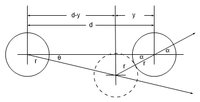The dashed ball shows the strike after aiming the cue off center angle $$\displaystyle \theta$$ showing the object ball moving at angle $$\displaystyle \theta$$ as a result. The equation relating $$\displaystyle \theta$$ and $$\displaystyle \alpha$$ is $$\displaystyle \sin\alpha = (\frac d D -\cos\alpha)\tan\theta$$, where $$\displaystyle D=2r$$ is the diameter of the ball. This is nonlinear. The plot below shows what it looks like using pool ball radius 2.5 inches and distance between the balls 20 inches.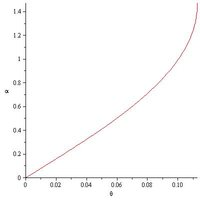##### New member
Thanks again, LCKurtz. I'm trying to follow. Did you mean for the x values to show from 0 to 0.10? When looking at the y axis, I had first thought you were using radians because of the 0-1.4 range.

So this equation covers the nonlinear relationship between θ and α for the same distance and diameter, but was I correct in assuming that the relationship among all angles in the θ range (from 0 degrees to whatever the upper extreme becomes as θ drops and that range compresses) remains a linear one?

#### LCKurtz

##### Full Member
Both axes are in radians. The scales on the two axes are different so you can see the graph nicely. You can tell from the graph that the relationship between $$\displaystyle \theta$$ and $$\displaystyle \alpha$$ is nearly linear for small cue angles $$\displaystyle \theta$$. But for larger cue angles and more severe cut shots the angle $$\displaystyle \alpha$$ changes more dramatically (curved portion of the graph). And, of course, for large cue angles you miss the object ball (not shown in the graph).

Yes. Sorry for changing the $$\displaystyle \theta$$ angle. I didn't notice I had done that when preparing the drawings. I play pool a bit myself so I found it interesting too. We calculated in the earlier post that the max value of $$\displaystyle \alpha$$, which I had earlier called $$\displaystyle \theta$$, is $$\displaystyle \alpha_{max} = \text{arccos}\left (\frac D d\right )$$. The max cue angle $$\displaystyle \theta$$ after which the cue ball misses the object ball is $$\displaystyle \theta_{max} = \frac \pi 2 - \alpha_{max} = \text{arcsin}\left ( \frac D d \right )$$. For our example where $$\displaystyle d=20$$ these come out $$\displaystyle \alpha_{max} = 83.54055018^\circ$$, $$\displaystyle \theta_{max} = 6.459449805^\circ$$ and sum to $$\displaystyle 89.99999998^\circ$$.
•